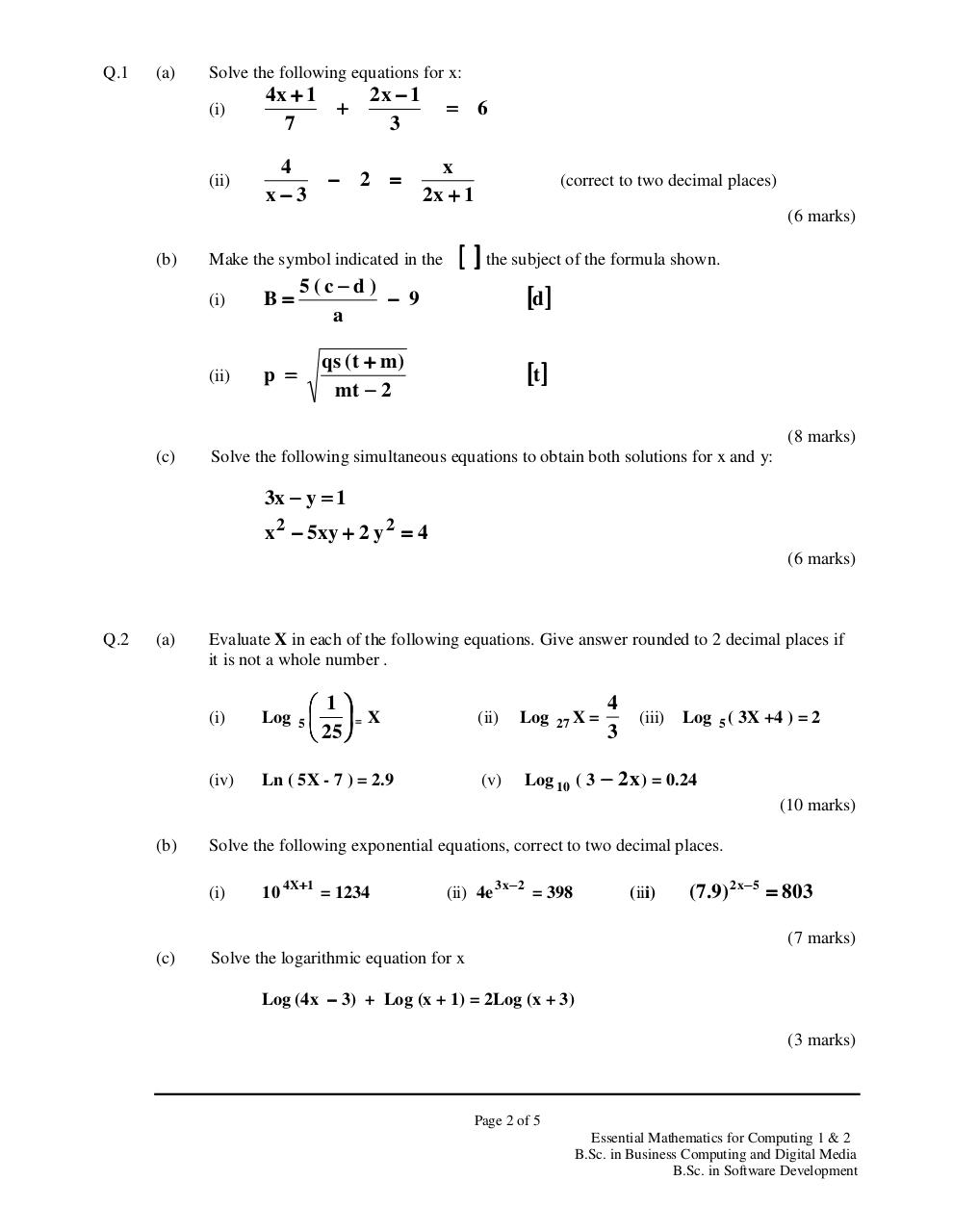# Maths (Summer 2010).PDFPage 1 2 3 4 5 6

#### Text preview

Q.1

(a)

Solve the following equations for x:
(i)

4x + 1
+
7

(ii)

4
x−3

2x − 1
3

− 2 =

= 6
x
2x + 1

(correct to two decimal places)
(6 marks)

(b)

Make the symbol indicated in the
(i)

B=

5(c−d )
− 9
a

(ii)

p =

qs ( t + m )
mt − 2

[ ] the subject of the formula shown.
[d]
[t ]
(8 marks)

(c)

Solve the following simultaneous equations to obtain both solutions for x and y:

3x − y = 1
x 2 − 5xy + 2 y 2 = 4
(6 marks)

Q.2

(a)

Evaluate X in each of the following equations. Give answer rounded to 2 decimal places if
it is not a whole number .

 1 
 = X
 25 

(i)

Log

(iv)

Ln ( 5X - 7 ) = 2.9

5

27 X

=

4
3

(ii)

Log

(v)

Log 10 ( 3 − 2x ) = 0.24

(iii)

Log 5 ( 3X +4 ) = 2

(10 marks)
(b)

Solve the following exponential equations, correct to two decimal places.
(i)

10

4 X+1

= 1234

(ii) 4e

3 x− 2

= 398

(iii)

( 7.9) 2 x− 5 = 803
(7 marks)

(c)

Solve the logarithmic equation for x
Log (4x − 3) + Log (x + 1) = 2Log (x + 3)
(3 marks)

Page 2 of 5
Essential Mathematics for Computing 1 &amp; 2
B.Sc. in Business Computing and Digital Media
B.Sc. in Software Development